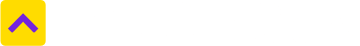Don't Miss out! Get notified of new homes as soon as they are posted

Notifications can be turned off anytime from browser settings.What is an EMI? How is it calculated?

If you are planning to get a home loan, the EMI or the equated monthly installments will be your main consideration. EMI is the sum of money that you as a borrower will pay your lender to clear your outstanding loan. These payments are made every month on a date that is stipulated by your bank till such time that the loan has been completely repaid. The three things that go into the calculation of an EMI are:

1) The amount of the loan

2) The rate of interest

3) The tenure of loan

The most popular method of computation is that of ‘monthly reducing loans’. In the monthly reducing cycle, the principal is reduced with every EMI and the interest is calculated on the balance outstanding. The majority of the retails such as Home loans, auto loans and personal loans are computed on a monthly reducing basis.

Effectively, therefore, in the initial years of the loan, a major component of the EMI is the interest that is payable by you. As the loan tenure reduces, the interest component reduces too, as the principal gets paid. Mathematically the following convention is applied for the calculation of an EMI:

Home loan EMI =

(Lxi) X (1+i) ^N / { (1+i) ^N}-1

L=Loan amount

i=Interest Rate (rate per annum divided by 12)

^= to the power of

N=loan period in months

(It is to be noted that an increase in the tenure of the loan will lead to an increase in interest rates and therefore, the interest component of your loan. As a borrower, you should try and pay as much of EMI as possible and shorten the tenure of the loan).